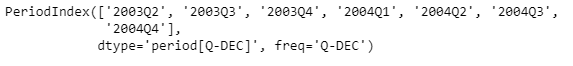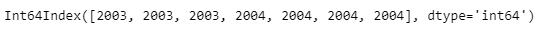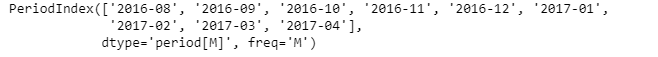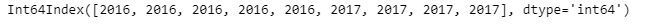Related Articles
Python | Pandas PeriodIndex.qyear
• Last Updated : 06 Jan, 2019

Python is a great language for doing data analysis, primarily because of the fantastic ecosystem of data-centric python packages. Pandas is one of those packages and makes importing and analyzing data much easier.

Pandas` PeriodIndex.qyear` attribute return an Index object containing the fiscal year the Period lies in according to its starting-quarter. The year and the qyear of the period will be the same if the fiscal and calendar years are the same. When they are not, the fiscal year can be different from the calendar year of the period.

Syntax : PeriodIndex.qyear

Parameters : None

Return : Index object

Example #1: Use `PeriodIndex.qyear` attribute to find out the fiscal year the period lies in for each period in the given PeriodIndex object.

 `# importing pandas as pd ` `import` `pandas as pd ` ` `  `# Create the PeriodIndex object ` `pidx ``=` `pd.PeriodIndex(start ``=``'2003-4-21 08:45 '``, ` `             ``end ``=``'2004-11-21 8:55'``, freq ``=``'Q'``) ` ` `  `# Print the PeriodIndex object ` `print``(pidx) `

Output :Now we will use the `PeriodIndex.qyear` attribute to find out the fiscal year of each period in pidx.

 `# return the fiscal year of the period ` `pidx.qyear `

Output :As we can see in the output, the `PeriodIndex.qyear` attribute has returned an Index object containing the fiscal year of each period for the given PeriodIndex object.

Example #2: Use `PeriodIndex.qyear` attribute to find out the fiscal year the period lies in for each period in the given PeriodIndex object.

 `# importing pandas as pd ` `import` `pandas as pd ` ` `  `# Create the PeriodIndex object ` `pidx ``=` `pd.PeriodIndex(start ``=``'2016-8-12 11:32'``,  ` `            ``end ``=``'2017-04-05 11:55'``, freq ``=``'M'``) ` ` `  `# Print the PeriodIndex object ` `print``(pidx) `

Output :Now we will use the `PeriodIndex.qyear` attribute to find out the fiscal year of each period in pidx.

 `# return the fiscal year of the period ` `pidx.qyear `

Output :As we can see in the output, the `PeriodIndex.qyear` attribute has returned an Index object containing the fiscal year of each period for the given PeriodIndex object.

Attention geek! Strengthen your foundations with the Python Programming Foundation Course and learn the basics.

To begin with, your interview preparations Enhance your Data Structures concepts with the Python DS Course.

My Personal Notes arrow_drop_up
Recommended Articles
Page :